Courses

# Design Of Power Screws Mechanical Engineering Notes | EduRev

## Mechanical Engineering : Design Of Power Screws Mechanical Engineering Notes | EduRev

The document Design Of Power Screws Mechanical Engineering Notes | EduRev is a part of the Mechanical Engineering Course Machine Design.
All you need of Mechanical Engineering at this link: Mechanical Engineering

Stresses in power screws

Design of a power screw must be based on the stresses developed in the constituent parts. A power screw is subjected to an axial load and a turning moment. The following stresses would be developed due to the loading:

a) Compressive stress is developed in a power screw due to axial load. Depending on the slenderness ratio it may be necessary to analyze for buckling. The compressive stress σc is given by σ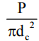here dc is the core diameter and if slenderness ratio λ is more than 100 or so buckling criterion must be used. λ is defined as λ =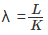where I=Ak2 and L is the length of the screw. Buckling analysis yields a critical load Pc and if both ends are assumed to be hinged critical load is given by PC =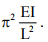In general the equation may be written as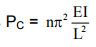where n is a constant that depends on end conditions.

b) Torsional shear stress is developed in the screw due to the turning moment and this is given by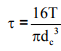where T is the torque applied

c) Bending stresses are developed in the screw thread and this is illustrated in figure-6.2.1.1. The bending moment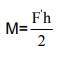and the bending stress on a single thread is given by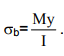Here y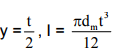and F′ is the load  on a single thread. Figure-6.2.1.2 shows a developed thread and figure shows a nut and screw assembly. This gives the bending stress at the thread root to be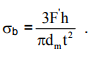This is clearly the most probable place for failure. Assuming that the load is equally shared by the nut threads

d) Bearing stress σbr at the threads is given by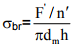e) Again on similar assumption shear stress τ at the root diameter is given by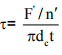Here n/ is the number of threads in the nut. Since the screw is subjected to torsional shear stress in addition to direct or transverse stress combined effect of bending, torsion and tension or compression should be considered in the design criterion.

Design procedure of a Screw Jack

A typical screw jack is shown in figure-6.2.2.1 . It is probably more informative to consider the design of a jack for a given load and lift. We consider a reasonable value of the load to be 100KN and lifting height to be 500mm. The design will be considered in the following steps:

1. Design of the screw

A typical screw for this purpose is shown in figure-6.2.2.2. Let us consider a mild steel screw for which the tensile and shear strengths may be taken to be approximately 448MPa and 224 MPa respectively. Mild steel being a ductile material we may take the compressive yield strength to be also close to 448MPa. Taking a very high factor of safety of 10 due to the nature of the application and considering the axial compression the core diameter of the screw dc is given by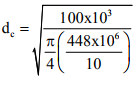which gives dc ≈ 54 mm. From the chart of normal series square threads in table- 6.1.1.1 the nearest standard nominal diameter of 70 mm is chosen, with pitch p = 10 mm. Therefore, core diameter dc = 60 mm , Major diameter dmaj = 70mm , Mean diameter dm = 65 mm , Nominal diameter dn = 70mm. The torque required to raise the load is given by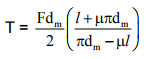Where l = np, n being the number of starts. Here we have a single start screw and hence l = p =10mm, dm = 65mm, F = 100X103 N Taking a safe value of μ for this purpose to be 0.26 and substituting the values we get T = 1027 Nm.

Check for combined stress

The screw is subjected to a direct compressive stress σc and a torsional shear stress τ. The stresses are given by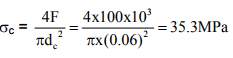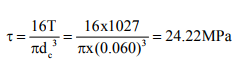The principal stress can be given by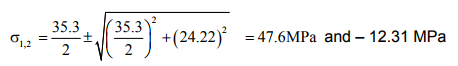and maximum shear stress τmax = 29.96 MPa.

The factor of safety in compression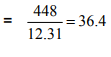and in shear =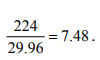Therefore the screw dimensions are safe. Check for buckling and thread stress are also necessary. However this can be done after designing the nut whose height and number of threads in contact is needed to determine the free length of the screw.

2. Design of the nut

A suitable material for the nut, as shown in figure- 6.2.2.3, is phosphor bronze which is a Cu-Zn alloy with small percentage of Pb and the yield stresses may be taken as

Yield stress in tension σty = 125MPa
Yield stress in compression σcy = 150MPa
Yield stress in shear τy = 105MPa
Safe bearing pressure Pb = 15MPa.

Considering that the load is shared equally by all threads bearing failure may be avoided if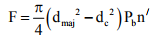where n/ is the number of threads in contact. Substituting values in the above equation we have n/ = 6.52. Let n/ =8. Therefore H = n/ p = 8X10 = 80mm. The nut threads are also subjected to crushing and shear. Considering crushing failure we have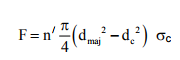This gives σc = 12.24 MPa which is adequately safe since σcy = 150 MPa and therefore crushing is not expected. To avoid shearing of the threads on the nut we may write F = πdmaj t n/ τ where t is the thread thickness which for the square thread is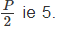ie 5. This gives τ =11.37 MPa and since τy= 105MPa shear failure of teeth is not expected. Due to the screw loading the nut needs to be checked for tension also and we may write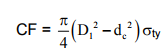A correlation factor C for the load is used to account for the twisting moment. With C=1.3 and on substitution of values in the equation D1 works out to be 70mm. But D1 needs to be larger than dmaj and we take D1 = 100mm. We may also consider crushing of the collar of the nut and to avoid this we may write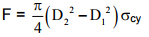Substituting values we have D2 = 110 mm. To allow for the collar margin we take D2 =120mm. Considering shearing of the nut collar πD1y = F . Substituting values we have a = 4mm Let a = 15mm

3. Buckling of the Screw.

Length L of the screw = Lifting height + H. This gives L= 500+80 = 580 mm With the nominal screw diameter of 70mm ,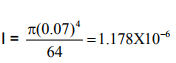and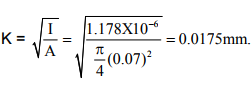The slenderness ration λ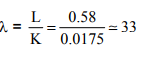This value of slenderness ratio is small (< 40) and the screw may be treated as a short column . No buckling of the screw is therefore expected.

4. Tommy bar

A typical tommy bar for the purpose is shown in figure-6.2.2.4.a. Total torsional moment without the collar friction is calculated in section 6.2.2.1 and T = 1027 Nm. The collar friction in this case ( see figure-6.2.2.1) occurs at the interface I. However in order to avoid rotation of the load when the screw rotates a loose fitting of the cup is maintained. Length l/ of the tommy bar = l1 + l3 and we may write the torque T as D3 T= F1l/ Where F1 is the maximum force applied at the tommy bar end and this may be taken as approximately 400 N . This gives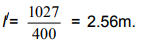This length of the tommy bar is too large and one alternative is to place the tommy bar centrally and apply force at both the ends. This alternative design of the tommy bar is also shown in figure-6.2.2.4.b The bar is subjected to a bending moment and its maximum value may be taken as 1027 Nm. This means to avoid bending we may write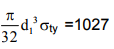where d1 is the tommy bar diameter as shown in figure- 6.2.2.4.b If we choose a M.S bar of σty = 448MPa the tommy bar diameter d1 works out to be d1 = 0.0285m. Let d1 = 30 mm and we choose d2 = 40mm

5. Other dimensions

D= (1.5 to 1.7 ) d           Let D= 112 mm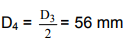Let L1 = 100 mm and t4 = 10 mm

t1 = 0.25 dn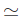18 mm , D52.25 D2 = 270 mm, D6 = 1.75 D5 = 473 mm, t3 = t1/2 = 9 mm.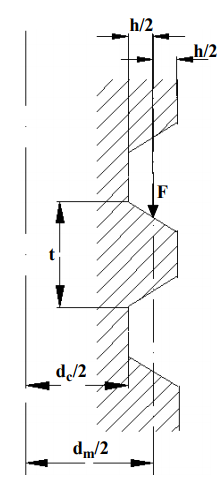6.2.1.1 F- Loading and bending stresses in screw threads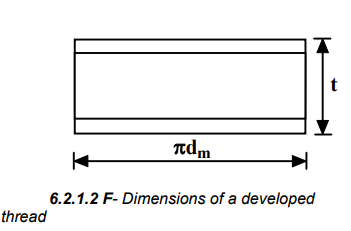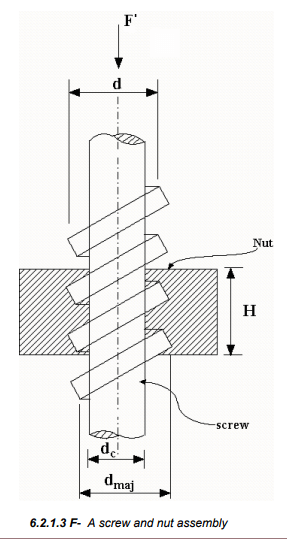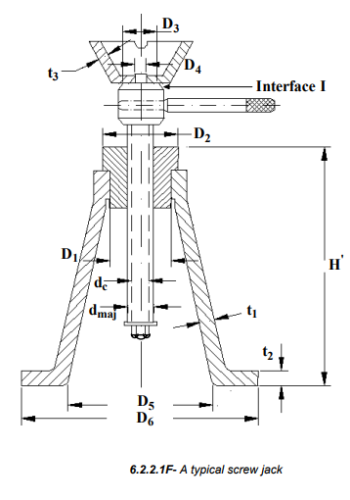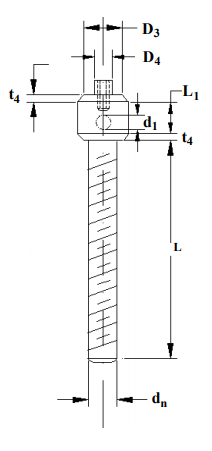6.2.2.2F- The screw with the provision for tommy bar attachment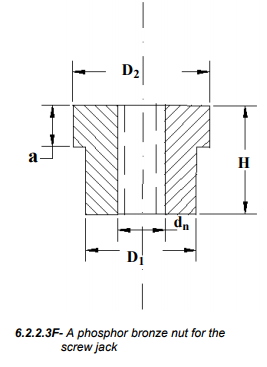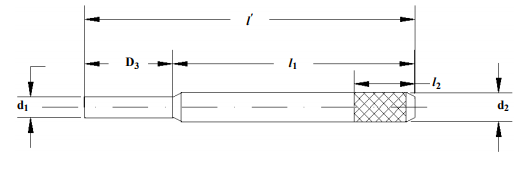6.2.2.2F- The screw with the provision for tommy bar attachment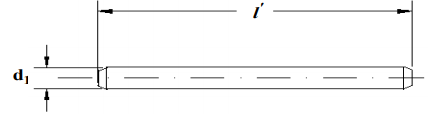6.2.2.4.b F- A typical centrally located tommy bar

Offer running on EduRev: Apply code STAYHOME200 to get INR 200 off on our premium plan EduRev Infinity!

## Machine Design

57 videos|71 docs|102 tests

,

,

,

,

,

,

,

,

,

,

,

,

,

,

,

,

,

,

,

,

,

;